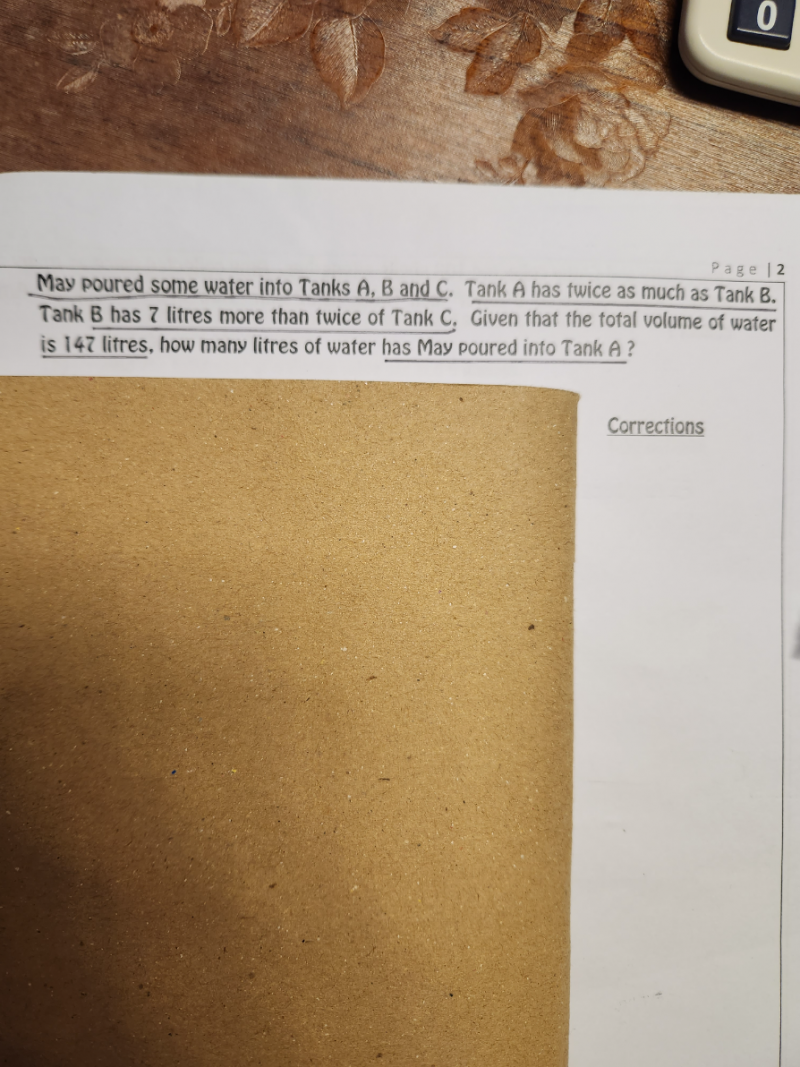# QuestionI don’t quite get the sentence on “Tank B has 7litres more than twice of Tank C”. Anyone can help with this question?

Since Tank C has the smallest amount of water, let u be the amount of water in Tank C.

Tank  C     [u]

Tank B      [u][u]

Tank A      [u][u][u][u]

Total = 147

From the model diagram,

7u + 21 = 147

u = 126/7  = 18

Hence Tank A has 4u+14 = 4(18)+14 = 86 litres

0 Replies 3 Likes ✔Accepted Answer

Thank you! I got it!

0 Replies 1 Like